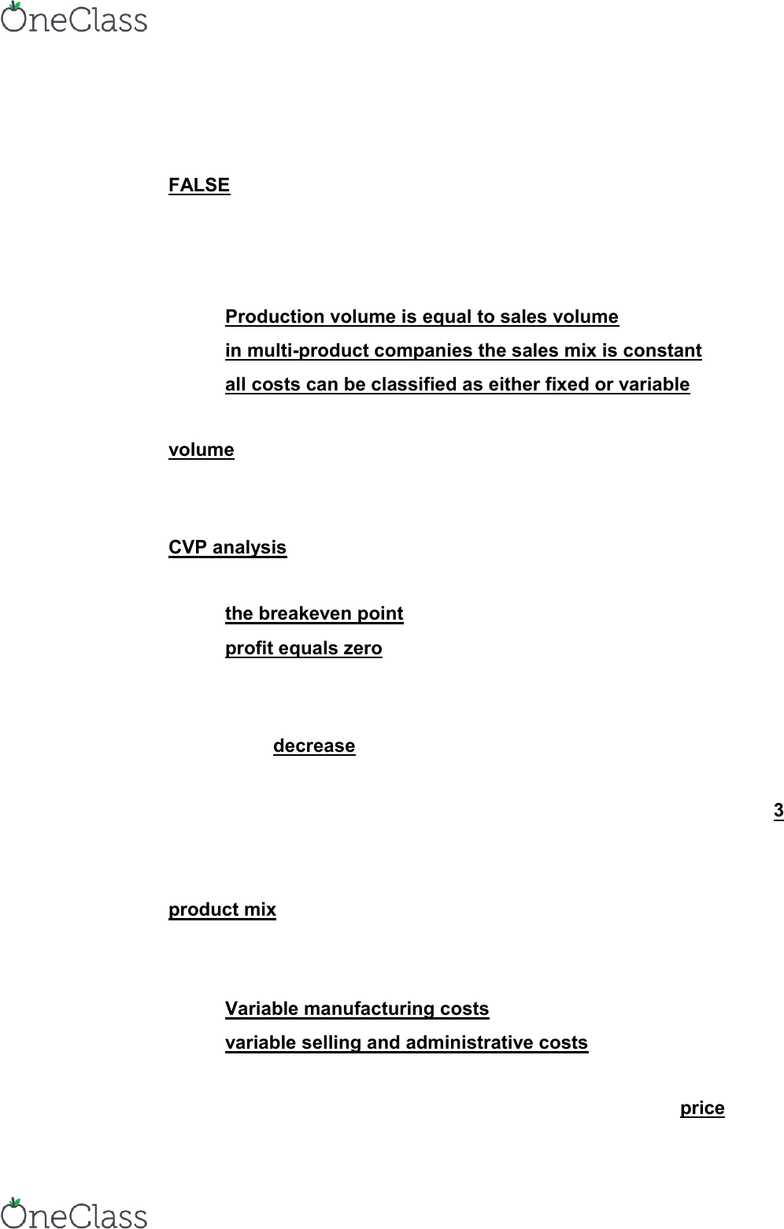Class Notes (1,100,000)
US (480,000)
LSU (7,000)
ACCT (100)
Lecture 6

# ACCT 2101 Lecture Notes - Lecture 6: Contribution Margin, Earnings Before Interest And Taxes, Tdw

Department
Accounting
Course Code
ACCT 2101
Professor
juliechenier
Lecture
6

Page:
of 7LearnSmart Chapter 6: Cost-Value Profit Analysis
TRUE/ FALSE
1. CVP Analysis is only used for breakeven and target profit analysis:
FALSE
MULTIPLE CHOICE
2. Which of the following are assumptions of cost volume profit analysis?:
Production volume is equal to sales volume
in multi-product companies the sales mix is constant
all costs can be classified as either fixed or variable
3. When constructing a CVP graph, the horizontal (x) axis represents unit of
volume
4. Which tool can be used to easily calculate the changes in profit resulting
from a change in sales price, sales volume, variable costs, or fixed cost?:
CVP analysis
5. CVP Graph indicates:
the breakeven point
profit equals zero
6. When a company increases the selling price of a product with no change
in variable cost per unit or total fixed costs, the breakeven point for that
product will: decrease
7. A firm that has a net operating income of \$50,000, a contribution margin of
\$150,000, and sales of \$300,000 has a degree of operating leverage of: 3
150,000/50,000=3
8. When multiple products are sold, the breakeven point depends on the
product mix
9. Which of the following must be subtracted from sales to reach the
contribution margin?:
Variable manufacturing costs
10. According to the assumptions of CVP, which of the following will NOT
change as the volume of a product increases or decreases?: price
find more resources at oneclass.com
find more resources at oneclass.com
LearnSmart Chapter 6: Cost-Value Profit Analysis
11. A company sells a product for \$80 per unit and has a contribution margin
ratio of 45%. Fixed costs total \$180,000. Sales dollar to breakeven equals:
\$400,000
\$180,000/0.45=400,000
12. Multi-product target profit analysis:
is calculated the same way as single product analysis
depends upon the sales mix
13. A company sells its products for \$40 per unit. Variable costs are \$12 per
unit. Total fixed costs are \$50,000. In order to reach their profit goal of
\$90,000, the company must sell 5,000 units of the product.
(50,000+90,000)/(40-12)=5,000 units
14. Jump-It Corporation has a margin of safety of \$272,000. The company
sold 100,000 units this year. If the company sells each unit for \$17, what is
the margin of safety percentage?: 16%
272,000/(100,000x17)=16%
15. The weighted average unit contribution margin:
assumes that the percentage of each product sold is constant
is used instead of the single product contribution margin
is multi-product breakeven analysis
is based on the relevant percentage of each unit sold
16. A company has fixed costs of \$25,000 and a weighted average unit
contribution margin of \$25. Of the sales, 65% are product XYZ and 35%
are product TDW. In order to breakeven the company must sell 650 units
of product XYZ and 350 units of product TDW
\$25,000/\$25=\$1,000x0.65=650
\$25,000/\$25=\$1,000x0.35=350
17. Which of the following is the equation used to calculate the degree of
operating leverage?:
Degree of operating leverage=Contribution margin/net
operating income
find more resources at oneclass.com
find more resources at oneclass.com
LearnSmart Chapter 6: Cost-Value Profit Analysis
18. Decisions about whether to use fixed or variable costs to run a business
impact a company’s: operating leverage
19. The equation used to calculate margin of safety in dollars is: Budgeted
(or actual) sales minus break-even sales
20. Steel, Inc. has a margin of safety in dollars of \$559,740. If actual sales
were \$2,946,000, Steel’s margin of safety percentage is 19%
\$559,740/\$2,946,000=19%
21. Jacki’s Jewels sells 10,000 necklace & earring sets per year. Her fixed
costs are \$80,000 and variable costs are \$20 per set. Jacki is planning to
increase the quality of the stones which will increase variable costs by \$8
per set. She expects that sales will increase by 25%. If Jacki increases the
quality of the stones, what price will she need to charge to attain her target
profit of \$60,000 per year for this set?: \$39.20
Cost+Target Profit=
(\$28+\$80,000+\$60,000)=\$490,000/(10,000x1.25)=\$39.20
22. In order to reach a target profit of \$180,000, 90,000 units need to be sold.
Assuming each unit sells for \$7.50, the total sales dollars needed to reach
the target profit is \$675,000
90,000x\$7.50=\$675,000
23. JVL Enterprises has set a target profit of \$126,000. The company sells a
single product for \$50 per unit. Variable costs are \$15 per unit and fixed
costs total \$98,000. How many units does JVL have to sell to break-
even?: \$2,800
\$98,000/(\$50-\$15)=2,800
24. Given fixed costs of \$30,000, variable costs of \$2.00 per unit, and a
contribution margin of \$5.00 unit, 6,000 units have to be sold in order to
break-even
\$30,000/\$5,000=6000 units
find more resources at oneclass.com
find more resources at oneclass.com